﻿ 一种新的圆锥误差优化补偿算法 A New Optimized Compensation Algorithm of Conic Error

Dynamical Systems and Control
Vol.07 No.01(2018), Article ID:23583,8 pages
10.12677/DSC.2018.71006

A New Optimized Compensation Algorithm of Conic Error

Lijun Gu, Xiaoning Fu

School of Electromechanical Engineering, Xidian University, Xi’an Shaanxi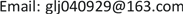Received: Dec. 22nd, 2017; accepted: Jan. 8th, 2018; published: Jan. 29th, 2018ABSTRACT

In order to solve the noncommutative error existing in the strap down inertial navigation system, this paper presents an improved coning error optimization algorithm to improve the solution accuracy. The algorithm optimizes the overlapped optimization algorithm further. Firstly, the error criterion of the classical coning motion is established; secondly, the optimized formula of the compensation algorithm is deduced and the optimal compensation coefficient is obtained; finally, the optimized algorithm under different coning motion environment is simulated. The results show that attitude calculation accuracy through improved algorithm is better than the traditional algorithm and overlapping algorithm; what’s more, the calculation accuracy of four-sample is better than the two-sample and three-sample.

Keywords:Strap-Down Inertial Navigation System, Equivalent Rotation Vector, Noncommutative Error, Compensation Coefficient1. 引言

2. 圆锥运动与圆锥误差

$a\left(t\right)/a={\left[\mathrm{cos}\omega t\text{\hspace{0.17em}}\text{\hspace{0.17em}}\mathrm{sin}\omega t\text{\hspace{0.17em}}\text{\hspace{0.17em}}0\right]}^{\text{T}}$ (1)

$q\left(t\right)=\left[\mathrm{cos}\left(a/2\right)\text{\hspace{0.17em}}\text{\hspace{0.17em}}\mathrm{sin}\frac{a}{2}\mathrm{cos}\omega t\text{\hspace{0.17em}}\text{\hspace{0.17em}}\mathrm{sin}\frac{a}{2}\mathrm{sin}\omega t\text{\hspace{0.17em}}\text{\hspace{0.17em}}0\right]$ (2)

$\omega \left(t\right)=\left[-\omega \mathrm{sin}a\mathrm{sin}\gamma t\text{\hspace{0.17em}}\text{\hspace{0.17em}}\omega \mathrm{sin}a\mathrm{cos}\omega t\text{\hspace{0.17em}}\text{\hspace{0.17em}}-2\omega {\mathrm{sin}}^{2}\frac{a}{2}\right]$ (3)

3. 优化算法的误差准则

$q\left(t+\Delta t\right)=q\left(t\right)\circ q\left(\Delta t\right)$ (4)

$q\left(\Delta t\right)={\left[\mathrm{cos}\frac{\Delta \sigma }{2}\text{\hspace{0.17em}}\text{\hspace{0.17em}}\frac{\Delta \sigma }{\Delta \sigma }\mathrm{sin}\frac{\Delta \sigma }{2}\right]}^{\text{T}}$ (5)

$\Delta \stackrel{¯}{\sigma }\approx \theta +\frac{1}{2}{\int }_{t}^{t+\Delta t}\theta \left(\tau \right)×\omega \text{d}\tau$ (6)

$\Delta \stackrel{¯}{\sigma }\text{=}\left[\begin{array}{l}\Delta {\stackrel{¯}{\sigma }}_{x}\\ \Delta {\stackrel{¯}{\sigma }}_{y}\\ \Delta {\stackrel{¯}{\sigma }}_{z}\end{array}\right]=\left[\begin{array}{c}-2\left(\mathrm{sin}\frac{\omega h}{2}+\left(-\omega h\mathrm{cos}\frac{\omega h}{2}+2\mathrm{sin}\frac{\omega h}{2}\right){\mathrm{sin}}^{2}\frac{a}{2}\right)\mathrm{sin}a\mathrm{sin}\omega \left(t+\frac{h}{2}\right)\\ 2\left(\mathrm{sin}\frac{\omega h}{2}+\left(-\omega h\mathrm{cos}\frac{\omega h}{2}+2\mathrm{sin}\frac{\omega h}{2}\right){\mathrm{sin}}^{2}\frac{a}{2}\right)\mathrm{sin}a\mathrm{cos}\omega \left(t+\frac{h}{2}\right)\\ -2\omega h{\mathrm{sin}}^{2}\frac{a}{2}+\frac{1}{2}{\mathrm{sin}}^{2}a\left(\omega h-\mathrm{sin}\omega h\right)\end{array}\right]$ (7)

$\begin{array}{l}\Delta \sigma ={\left[\Delta {\sigma }_{x}\text{\hspace{0.17em}}\text{\hspace{0.17em}}\Delta {\sigma }_{y}\text{\hspace{0.17em}}\text{\hspace{0.17em}}\Delta {\sigma }_{z}\right]}^{\text{T}}\\ \text{\hspace{0.17em}}\text{\hspace{0.17em}}\text{\hspace{0.17em}}\text{\hspace{0.17em}}=\underset{i=1}{\overset{N}{\sum }}{\theta }_{i}+\underset{i=1}{\overset{N-1}{\sum }}{K}_{i}\left({\theta }_{i}×{\theta }_{N}\right)+G\left({\theta }^{\prime }×\theta \right)\end{array}$ (8)

${\theta }_{i}×{\theta }_{N}=\left[\begin{array}{c}-8\frac{\omega h}{N}{\mathrm{sin}}^{2}\frac{a}{2}\mathrm{sin}a\mathrm{sin}\frac{\omega h}{2N}\mathrm{sin}\frac{N-i}{2N}\omega h\mathrm{sin}\omega \left(t+\frac{N+i-1}{2N}h\right)\\ 8\frac{\omega h}{N}{\mathrm{sin}}^{2}\frac{a}{2}\mathrm{sin}a\mathrm{sin}\frac{\omega h}{2N}\mathrm{sin}\frac{N-i}{2N}\omega h\mathrm{cos}\omega \left(t+\frac{N+i-1}{2N}h\right)\\ 4{\mathrm{sin}}^{2}a{\mathrm{sin}}^{2}\frac{\omega h}{2N}\mathrm{sin}\omega \left(N-i\right)\frac{h}{N}\end{array}\right]$ (9)

$\underset{i=1}{\overset{N}{\sum }}{\theta }_{i}=\left[\begin{array}{c}-2\mathrm{sin}a\mathrm{sin}\frac{\omega h}{2}\mathrm{sin}\omega \left(t+\frac{h}{2}\right)\\ 2\mathrm{sin}a\mathrm{sin}\frac{\omega h}{2}\mathrm{cos}\omega \left(t+\frac{h}{2}\right)\\ -2\omega h{\mathrm{sin}}^{2}\frac{a}{2}\end{array}\right]$ (10)

${\theta }^{\prime }×\theta =\left[\begin{array}{c}-8\omega h{\mathrm{sin}}^{2}\frac{a}{2}\mathrm{sin}a{\mathrm{sin}}^{2}\frac{\omega h}{2}\mathrm{sin}\omega t\\ 8\omega h{\mathrm{sin}}^{2}\frac{a}{2}\mathrm{sin}a{\mathrm{sin}}^{2}\frac{\omega h}{2}\mathrm{cos}\omega t\\ 4{\mathrm{sin}}^{2}a{\mathrm{sin}}^{2}\frac{\omega h}{2}\mathrm{sin}\omega h\end{array}\right]$ (11)

$\epsilon =|\Delta {\stackrel{¯}{\sigma }}_{x}-\Delta {\sigma }_{x}|$ (12)

$\begin{array}{c}\epsilon =A|\left(-\omega h\mathrm{cos}\frac{\omega h}{2}+2\mathrm{sin}\frac{\omega h}{2}\right)\mathrm{sin}\omega \left(t+\frac{h}{2}\right)\\ \text{\hspace{0.17em}}\text{\hspace{0.17em}}-4\frac{\omega h}{N}\mathrm{sin}\frac{\omega h}{2N}\underset{i=1}{\overset{N-1}{\sum }}{\lambda }_{i}\mathrm{sin}\frac{N-i}{2N}\omega h\mathrm{sin}\omega \left(t+\frac{N+i-1}{2N}h\right)\\ \text{\hspace{0.17em}}\text{\hspace{0.17em}}-4G\omega h{\mathrm{sin}}^{2}\frac{\omega h}{2}\mathrm{sin}\omega t|\end{array}$ (13)

$A=2{\mathrm{sin}}^{2}\frac{a}{2}\mathrm{sin}a$ (14)

4. 确定优化算法的系数

${\lambda }_{i}={\kappa }_{i}$ 时，式(8)是未进行优化的重叠式算法的表达式。对重叠式算法进行优化后的系数如表1所示。

1) 将式(13)中的正弦函数全都展开成关于 $\omega \left(t+\frac{h}{2}\right)$ 的三角函数，并对同类项进行合并；

2) 令关于 $\omega \left(t+\frac{h}{2}\right)$ 的余弦函数项的系数为0，得到：Table 1. Optimized coefficient of overlapping algorithm

$4\frac{\omega h}{N}\mathrm{sin}\frac{\omega h}{2N}\underset{i=1}{\overset{N-1}{\sum }}{\lambda }_{i}\mathrm{sin}\frac{N-i}{2N}\omega h\mathrm{sin}\frac{i-1}{2N}\omega h+4G\omega h{\mathrm{sin}}^{2}\frac{\omega h}{2}\mathrm{sin}\frac{\omega h}{2}=0$ (15)

3) 对下述公式中关于 $\omega \left(t+\frac{h}{2}\right)$ 的正弦函数项的系数X进行幂级数展开，即：

$\begin{array}{c}X=-\omega h\mathrm{cos}\frac{\omega h}{2}+2\mathrm{sin}\frac{\omega h}{2}-4\frac{\omega h}{N}\mathrm{sin}\frac{\omega h}{2N}\underset{i=1}{\overset{N-1}{\sum }}{\lambda }_{i}\mathrm{cos}\frac{i-1}{2N}\omega h\mathrm{sin}\frac{N-i}{2N}\omega h\\ \text{\hspace{0.17em}}\text{\hspace{0.17em}}-4G\omega h{\mathrm{sin}}^{2}\frac{\omega h}{2}\mathrm{cos}\frac{\omega h}{2}\\ =\left[\frac{1}{12}{\left(\omega h\right)}^{3}-\frac{1}{480}{\left(\omega h\right)}^{5}+\cdot \cdot \cdot \right]\\ \text{\hspace{0.17em}}\text{\hspace{0.17em}}-\underset{i=1}{\overset{N-1}{\sum }}{\lambda }_{i}\left(N-i\right)\left[{\left(\frac{\omega h}{N}\right)}^{3}-\frac{1+{\left(N-i\right)}^{2}+3{\left(i-1\right)}^{2}}{24}{\left(\frac{\omega h}{N}\right)}^{5}+\cdot \cdot \cdot \right]\\ \text{\hspace{0.17em}}\text{\hspace{0.17em}}-\left[G{\left(\omega h\right)}^{3}-G\frac{5}{24}{\left(\omega h\right)}^{5}+\cdot \cdot \cdot \right]\end{array}$ (16)

4) 对上述公式中的同类项进行合并，并且令与 ${\lambda }_{i}$ 有关且阶次不超过(2N − 3)的各项的系数均为0，即：

$\left\{\begin{array}{l}\frac{1}{12}-\underset{i=1}{\overset{N-1}{\sum }}{\lambda }_{i}\frac{N-i}{{N}^{3}}-G=0\\ \frac{1}{480}-\underset{i=1}{\overset{N-1}{\sum }}{\lambda }_{i}\frac{\left(N-i\right)\left[1+{\left(N-i\right)}^{2}+3{\left(i-1\right)}^{2}\right]}{24{N}^{5}}-\frac{5}{24}G=0\\ \text{\hspace{0.17em}}\text{\hspace{0.17em}}\text{\hspace{0.17em}}\text{\hspace{0.17em}}\text{\hspace{0.17em}}\text{\hspace{0.17em}}\text{\hspace{0.17em}}\text{\hspace{0.17em}}\text{\hspace{0.17em}}\text{\hspace{0.17em}}\text{\hspace{0.17em}}\text{\hspace{0.17em}}\text{\hspace{0.17em}}\text{\hspace{0.17em}}\text{\hspace{0.17em}}\text{\hspace{0.17em}}\text{\hspace{0.17em}}\text{\hspace{0.17em}}\text{\hspace{0.17em}}⋮\end{array}$ (17)

5) 根据式(15) (17)确定二次优化补偿系数 ${\lambda }_{i}$

5. 仿真验证

6. 结论Table 2. Optimized compensation coefficient of algorithm in this paper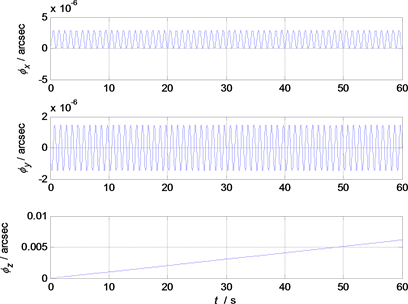Figure 1. Traditional algorithm attitude error of the two-sample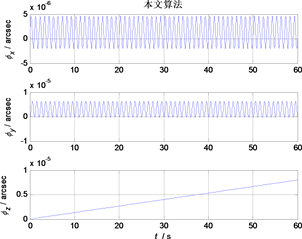Figure 2. Attitude error contrast of overlapping algorithm and algorithm of the two-sample in this paperFigure 3. Traditional algorithm attitude error of the three-sample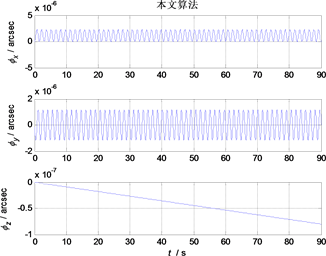Figure 4. Attitude error contrast of overlapping algorithm and algorithm of the three-sample in this paper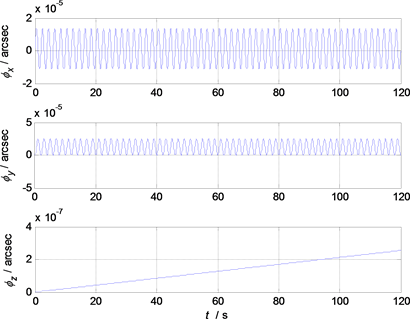Figure 5. Traditional algorithm attitude error of the four-sample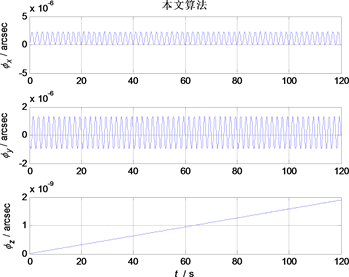Figure 6. Attitude error contrast of overlapping algorithm and algorithm of the four-sample in this paper

A New Optimized Compensation Algorithm of Conic Error[J]. 动力系统与控制, 2018, 07(01): 61-68. http://dx.doi.org/10.12677/DSC.2018.71006

1. 1. 李海涛, 曹咏弘, 祖静. 等效旋转矢量法在旋转弹姿态解算中的应用[J]. 测试技术学报, 2011, 25(4): 287-291.

2. 2. 程承, 潘泉, 李汉舟. 一种新的捷联惯导系统圆锥误差补偿算法研究[J]. 弹箭与制导学报, 2014, 34(1): 1-4.

3. 3. Bortz, J.E. (2007) A New Mathematical Formulation for Strapdown Inertial Navigation. IEEE Trans-actions on Aerospace & Electronic Systems, AES-7, 61-66.
https://doi.org/10.1109/TAES.1971.310252

4. 4. Jordan, J.W. (1969) An Accurate Strapdown Direction Cosine Algorithm.

5. 5. 秦永元, 张士邈. 捷联惯导姿态更新的四子样旋转矢量优化算法研究[J]. 中国惯性技术学报, 2001, 9(4): 1-7.

6. 6. 陈建锋, 陈熙源, 祝雪芬. 一种基于二次优化的改进圆锥算法[J]. 中国惯性技术学报, 2012, 20(2): 131-135.

7. 7. 丁杨斌, 满顺强, 申功勋. 一种新的捷联惯导系统圆锥误差补偿算法[J]. 北京航空航天大学学报, 2007, 33(11): 1261-1263.

8. 8. 肖胜. 激光惯导系统流水式旋转矢量算法研究[J]. 光学与光电技术, 2015, 13(4): 51-56.

9. 9. 王真, 高凤岐, 高敏, 等. 旋转矢量多迭代捷联姿态解算误差补偿算法[J]. 中国测试, 2016, 42(8): 113-117.

10. 10. 杨浩天, 汪立新, 王琪, 等. 一种改进的重叠式三子样圆锥误差补偿算法[J]. 电光与控制, 2017(3): 20-23.